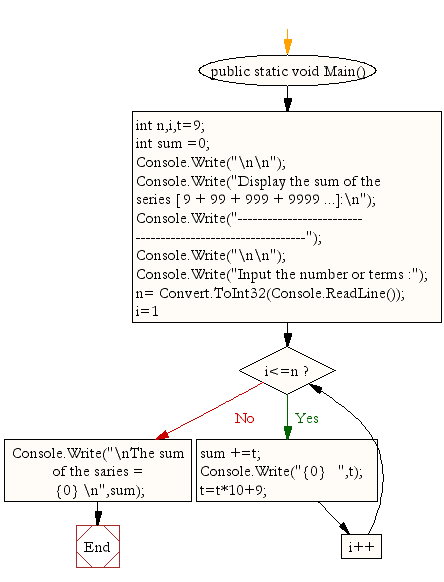﻿ C Sharp - Show the sum of the series [ 9 + 99 + 999 + 9999...]# C# Sharp Exercises: Display the sum of the series [ 9 + 99 + 999 + 9999 ...]

## C# Sharp For Loop: Exercise-21 with Solution

Write a program in C# Sharp to display the sum of the series [ 9 + 99 + 999 + 9999 ...].

Sample Solution:-

C# Sharp Code:

``````using System;
public class Exercise21
{
public static void Main()
{
int n,i,t=9;
int sum =0;

Console.Write("\n\n");
Console.Write("Display the sum of the series [ 9 + 99 + 999 + 9999 ...]:\n");
Console.Write("-----------------------------------------------------------");
Console.Write("\n\n");

Console.Write("Input the number or terms :");
for (i=1;i<=n;i++)
{ sum +=t;
Console.Write("{0}   ",t);
t=t*10+9;
}
Console.Write("\nThe sum of the saries = {0} \n",sum);
}
}
```
```

Sample Output:

```
Display the sum of the series [ 9 + 99 + 999 + 9999 ...]:
-----------------------------------------------------------
Input the number or terms :5
9   99   999   9999   99999
The sum of the saries = 111105
```

Flowchart:C# Sharp Code Editor:

Contribute your code and comments through Disqus.

What is the difficulty level of this exercise?

Test your Programming skills with w3resource's quiz.

﻿